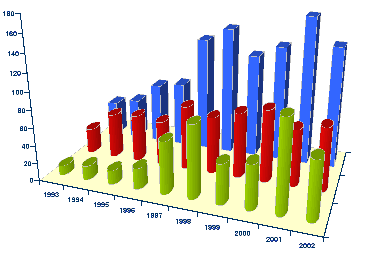DATA MINING
Desktop Survival Guide
by Graham Williams# Understanding DataA key task in any data mining project is exploratory data analysis (often abbreviated as EDA). This task generally involves getting the basic statistics of a dataset and using graphical tools to visually investigate the data's characteristics. Visual data exploration can help in understanding the data, in error correction, and in variable selection and variable transformation.

Statistics is the fundamental tool in understanding data. Statistics is essentially about uncertainty--to understand and thereby to make allowance for it. It also provides a framework for understanding the discoveries made in data mining. Discoveries need to be statistically sound and statistically significant--any uncertainty associated with the modelling needs to be understood.

Visualising data has been an area of study within statistics for many years. A vast array of tools are available for presenting data visually. The whole topic deserves a book in its own right, and indeed there are many, including () and Tufte.

In this chapter we introduce some of the basic statistical concepts that a data miner needs to know. We then provide a gallery of graphical approaches to visualise and understand our data. Many of the plots we present here could have just as easily, or perhaps initially even more easily, been produced using a spreadsheet application. However there are significant advantages in programmatically generating the plots. There could be tens, or even hundreds, of plots you would like to generate. Doing this by hand in a spreadsheet is cumbersome and error prone. Also, any plots produced from the first data extraction are just the start. As the data is refined and new datasets generated, manually regenerating plots is not a productive exercise. Using R to extract and manipulate the data and to plot the data is a cost effective exercise, using open source software (on either GNU/Linux or MSWindows platforms).

After loading data, as discussed in Chapter, we can start our exploration of the data itself. In addition to textual summaries, building on the basic graphics capabilities introduced in See Section 31, we provide an overview of R's extensive graphics capabilities for exploring and understanding the data. Section 32.1 explores the basic characteristics of a dataset, while Section 32.7 begins to provide basic statistical summaries of the data.

Subsections